Ex 9.3

Chapter 9 Class 11 Sequences and Series
Serial order wise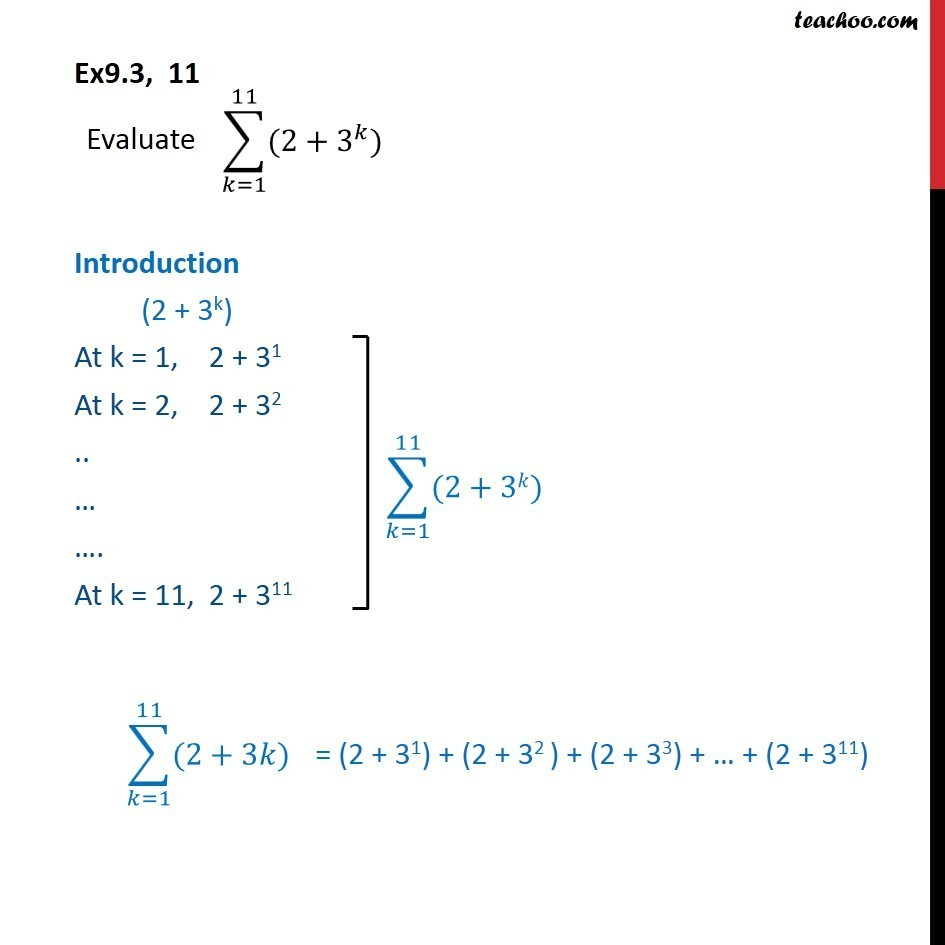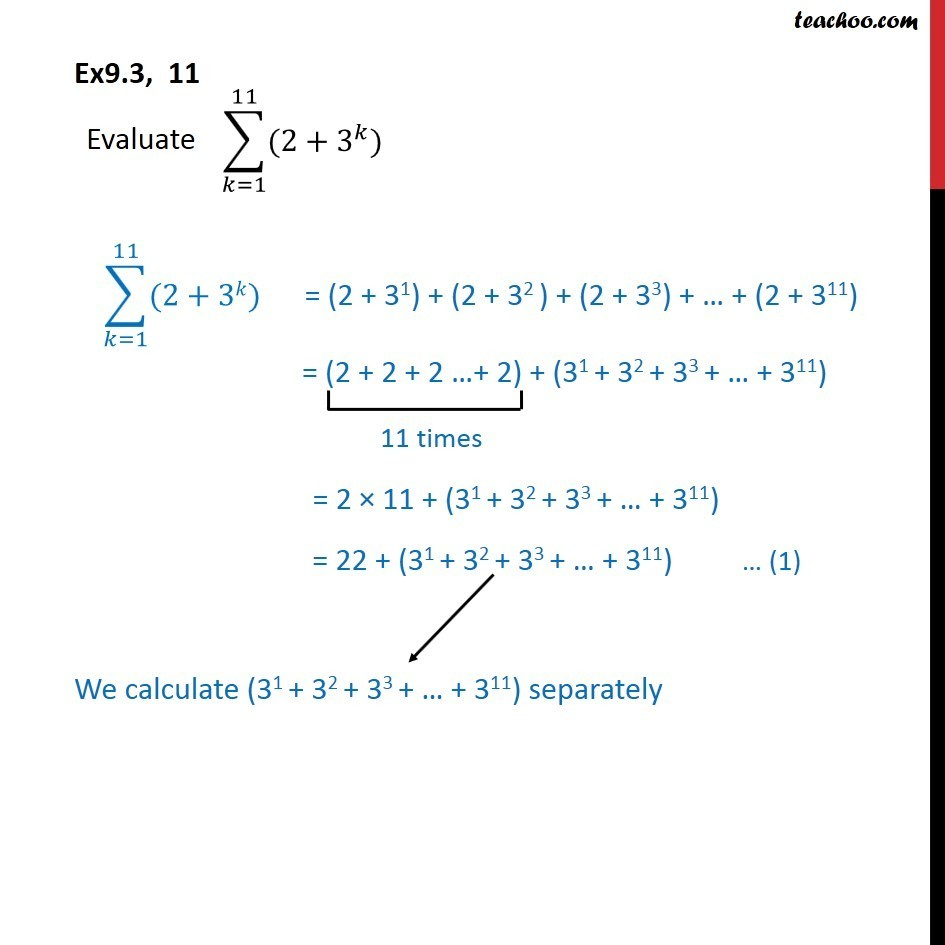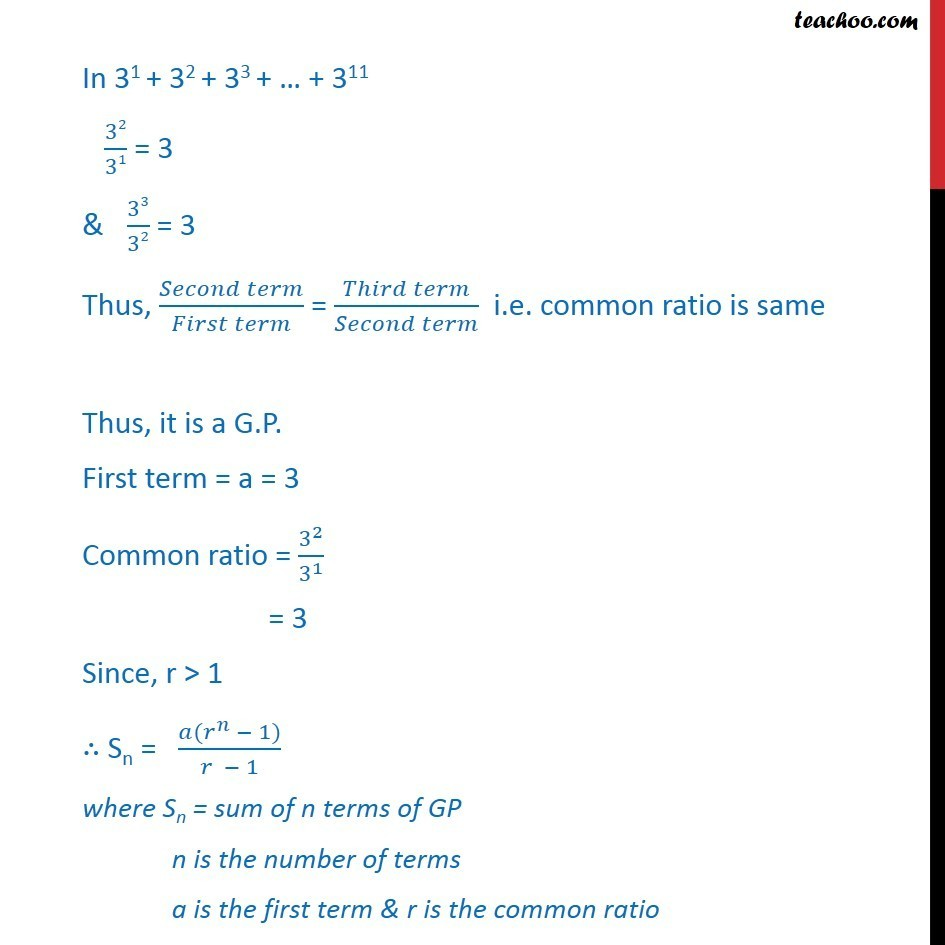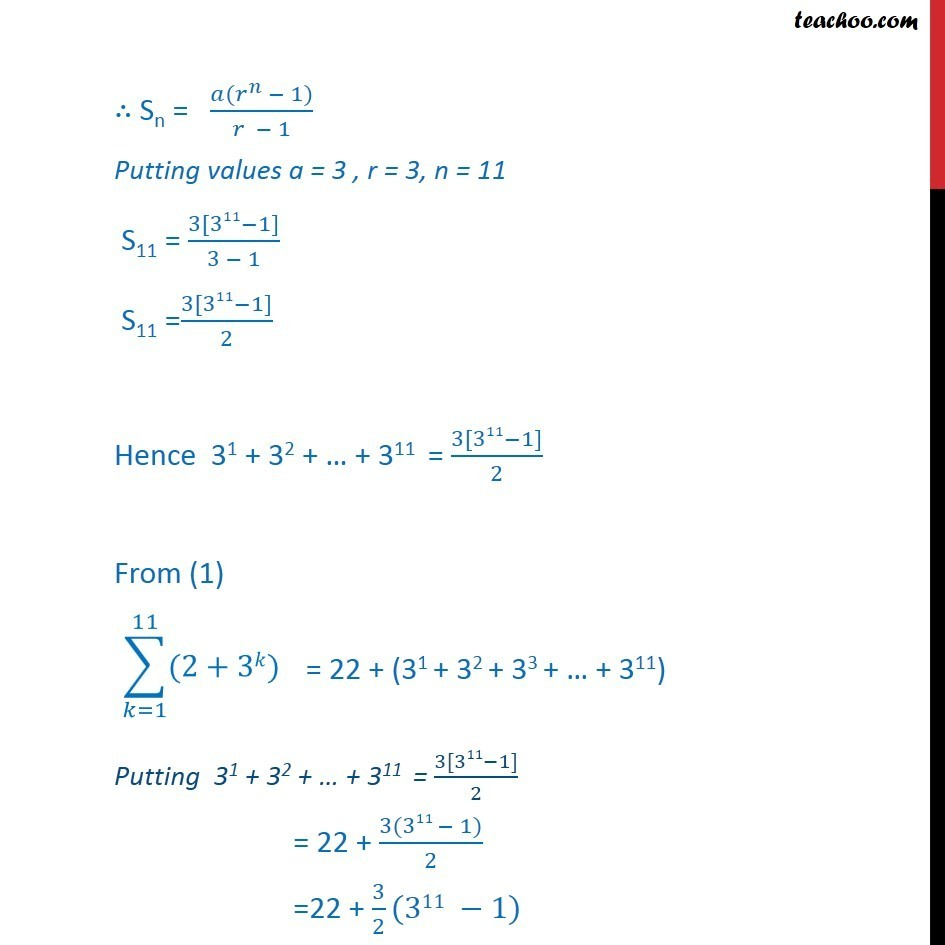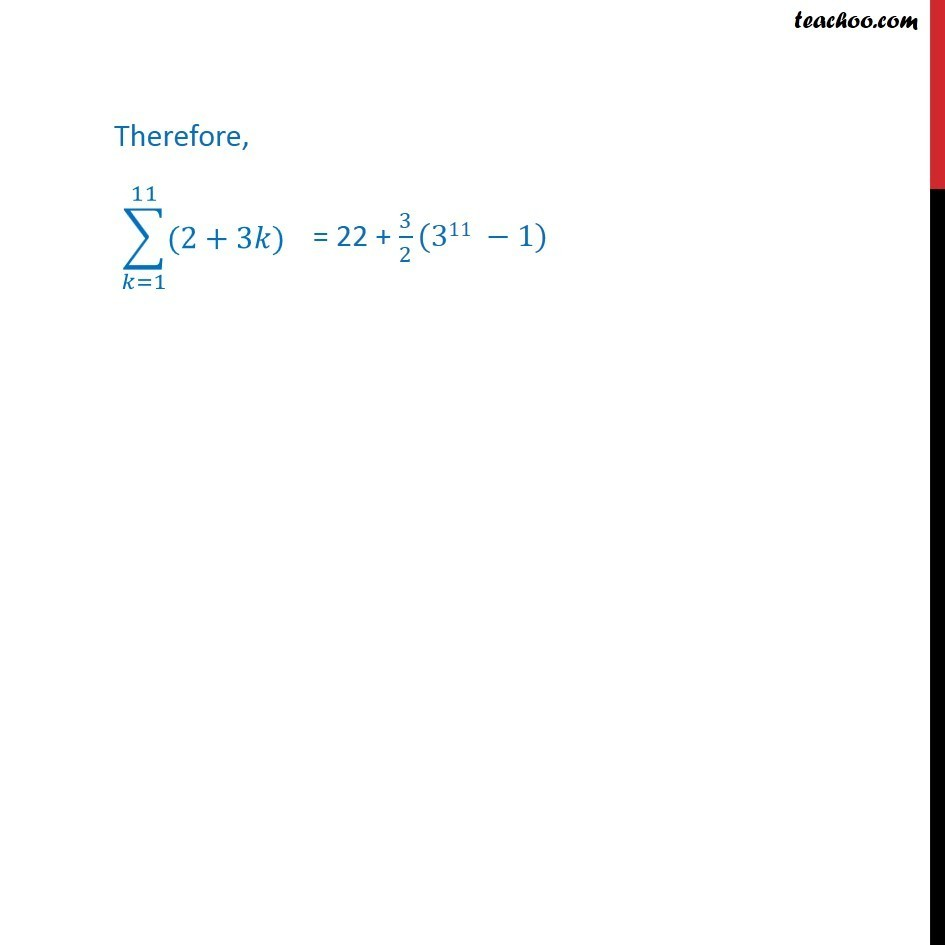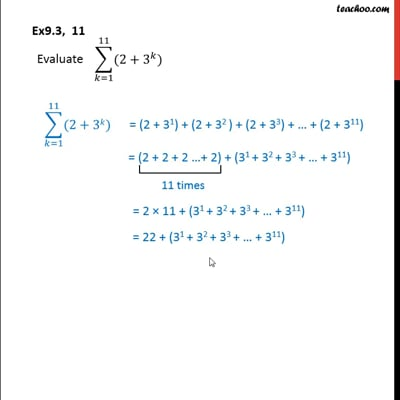This video is only available for Teachoo black users

Maths Crash Course - Live lectures + all videos + Real time Doubt solving!

### Transcript

Ex9.3, 11 Introduction (2 + 3k) At k = 1, 2 + 31 At k = 2, 2 + 32 .. … …. At k = 11, 2 + 311 Ex9.3, 11 We calculate (31 + 32 + 33 + … + 311) separately In 31 + 32 + 33 + … + 311 32/31 = 3 & 33/32 = 3 Thus, (𝑆𝑒𝑐𝑜𝑛𝑑 𝑡𝑒𝑟𝑚)/(𝐹𝑖𝑟𝑠𝑡 𝑡𝑒𝑟𝑚) = (𝑇ℎ𝑖𝑟𝑑 𝑡𝑒𝑟𝑚)/(𝑆𝑒𝑐𝑜𝑛𝑑 𝑡𝑒𝑟𝑚) i.e. common ratio is same Thus, it is a G.P. First term = a = 3 Common ratio = 3^2/3^1 = 3 Since, r > 1 ∴ Sn = (𝑎(𝑟^𝑛 − 1))/(𝑟 − 1) where Sn = sum of n terms of GP n is the number of terms a is the first term & r is the common ratio ∴ Sn = (𝑎(𝑟^𝑛 − 1))/(𝑟 − 1) Putting values a = 3 , r = 3, n = 11 S11 = (3[311−1])/(3 − 1) S11 =(3[311−1])/2 Hence 31 + 32 + … + 311 = (3[311−1])/2 From (1) Putting 31 + 32 + … + 311 = (3[311−1])/2 = 22 + (3(311 − 1))/2 =22 + 3/2(311 −1) Therefore,